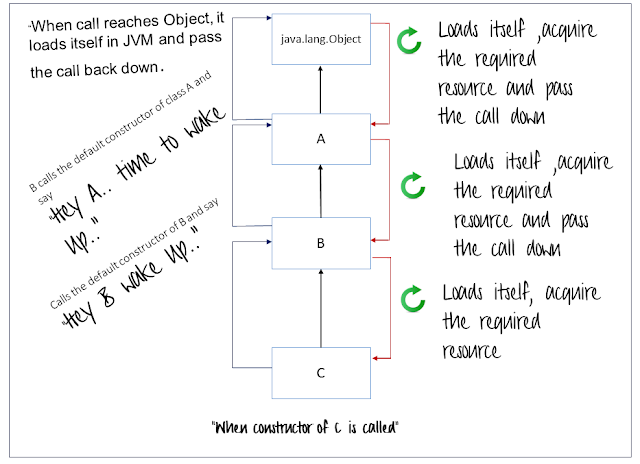WHAT'S NEW?

# Constructor Chaining

Whenever any sub class constructor is invoked , it by default invokes the default constructor of its super class, unless except parameterized constructor is explicitly invoked.

To understand the concept of constructor chaining let us see an example.

A.java
class A
{
int x=10;

public void m1()
{
System.out.println("m1-A()");
}

}

B.java

class B extends A

{
int y=20;

public void m2()
{
System.out.println("m2-B");
}

}

C.java

class C extends B

{

int z=20;

public void m3()
{
System.out.println("m3-C");
}

}

Demo.java

public class Demo

{
public static void main(String[] args)
{
C obj=new C();
System.out.println("X="+obj.x);
System.out.println("Y="+obj.y);
System.out.println("Z="+obj.z);
}
}Output
I have a simple question , never in the working did i called the object of A and B, so how come the values of x and y which belongs to A and B respectively got activated?

As a dead man cannot talk, a java class not loaded in JVM cannot provide the values. SO somewhere in JVM the classes A and B got loaded.

How?

Due to constructor chaining.

Lets see what happened. According to the chaining rule, whenever any sub class constructor is invoked it by default invokes the default constructor of its super class.
So when the class C constructor was called it called the constructor of class B.
When constructor of class B was called it called constructor of class A.
When constructor of class A was called it called constructor of java.lanag.Object.

In short whenever any sub class is called it wakes up all its super class in JVM.Constructor Chaining

- Hence when we call C() it calls B() in turn which calls A() and in turn it calls Object. Loads themselves in memory and get the required resources.

Now let us again write the example above with bit of modification.

A.java
class A
{
int x=10;
A()
{
System.out.println("Constructor A() called");
}
public void m1()
{
System.out.println("m1-A()");
}

}

B.java

class B extends A

{
int y=20;
B()
{
System.out.println("Constructor B() called");
}
public void m2()
{
System.out.println("m2-B");
}

}

C.java

class C extends B

{

int z=20;

C()
{
System.out.println("Constructor C() called");
}

public void m3()
{
System.out.println("m3-C");
}

}

Demo.java

public class Demo

{
public static void main(String[] args)
{
C obj=new C();
System.out.println("X="+obj.x);
System.out.println("Y="+obj.y);
System.out.println("Z="+obj.z);
}
}

Output

It must be very much clear now that the Constructor of super class are called before the sub class constructors are invoked.

But the rule is very simple, chaining works very simply only when the classes have default constructor. By now whatever we have seen just proves the first part where there are default constructor available in the class.

## What happens to constructor chaining when we do not have default constructor?

Let us again take another set of example to understand this scenario.
X.java

class X
{
int m=0;

X(int i)
{
System.out.println("X() with parameter is called");
}
public void m1()
{
System.out.println("m1-X");
}
}

Y.java

class Y extends X
{
int n=10;

Y()
{
System.out.println("Y() is called");
}
public void m2()
{
System.out.println("m2-Y");
}
}

Z.java

class Z extends Y
{
int o=100;

Z()
{
System.out.println("Z() is called");
}
public void m3()
{
System.out.println("m3-Z");
}
}

Demo.java

public class Demo
{
public static void main(String[] args)
{
Z obj=new Z();
System.out.println("M="+obj.m);
System.out.println("N="+obj.n);
System.out.println("O="+obj.o);
}
}

When compiled this program will give an error.

Here the chain got broken as X did not have a default constructor.

- But there is one way through which we can by pass this situation.
Calling the parameterized constructor using keyword super.

So we have to explicitly mention in the constructor of class Y that we are supposed to call the paramterized constructor of class X and not the default constructor of X.

## super keyword in an nutshell

super keyword is used to refer the object of its immediate super class.
As if we are accessing object of super class using the keyword super.

super can be used to access three members of class.

1. Variable

Let say class X have a variable m, we can access variable m using keyword super from class Y.

int j=super.m;
from somewhere in class Y.

2. Method

Let say class X have a method m1() , we can access m1() using keyword super from class Y.

super.m1();
from somewhere in class Y.

3. Constructor

Call to the constructor using super is bound with some rules.
Let say class X have a parameterized constructor taking an int variable,we can call it using keyword super,

1. Call to super() can be made only from subclass constructor and no other place.
2. Call to super should be the first executable line of code in the constructor.

So the constructor of Y would be edited like this.

Y()
{
super(10);
System.out.println("Y() is called");
}

## So back to Constructor Chaining

Now if we make above described changes to the constructor chaining will now be possible as earlier stating the other half of the rule that, unless except paramterized constructor has to be explicitly invoked.

So the revised classes will look like.

X.java

class X
{
int m=0;

X(int i)
{
System.out.println("X() with parameter is called");
}
public void m1()
{
System.out.println("m1-X");
}
}

Y.java

class Y extends X
{
int n=10;

Y()
{
super(5);
System.out.println("Y() is called");
}
public void m2()
{
System.out.println("m2-Y");
}
}

Z.java

class Z extends Y
{
int o=100;

Z()
{
System.out.println("Z() is called");
}
public void m3()
{
System.out.println("m3-Z");
}
}

Demo.java

public class Demo
{
public static void main(String[] args)
{
Z obj=new Z();
System.out.println("M="+obj.m);
System.out.println("N="+obj.n);
System.out.println("O="+obj.o);
}